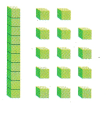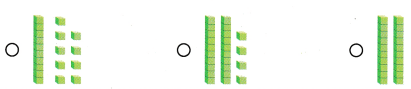# Texas Go Math Grade 1 Lesson 1.6 Answer Key Show Numbers in Different Ways

Refer to our Texas Go Math Grade 1 Answer Key Pdf to score good marks in the exams. Test yourself by practicing the problems from Texas Go Math Grade 1 Lesson 1.6 Answer Key Show Numbers in Different Ways.

## Texas Go Math Grade 1 Lesson 1.6 Answer Key Show Numbers in Different Ways

Essential Question
How can making a model help you show number in different ways?

Unlock the Problem
Gary and Jill both want 23 stickers for a class project. There are 3 sheets of 10 stickers and 30 single stickers on the table. How could Gary and Jill each take 23 stickers?

What information am I given?
The number is ___.
The number is 23

Plan
What is my plan or strategy?
I can _____
I can find the different ways to combine tens and ones with  base-ten blocks helps to share the stickers

Solve
Show how you solve the problem.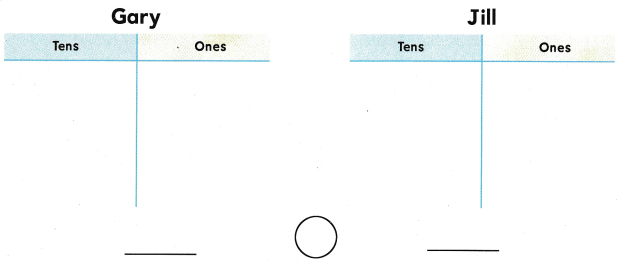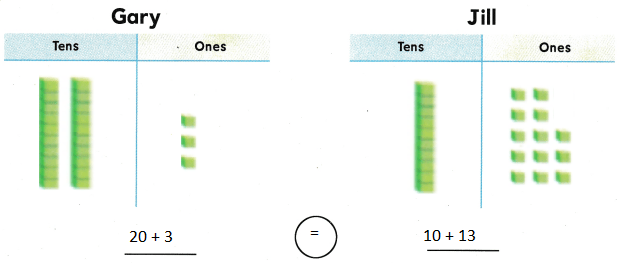Explanation:
Total number is blocks are 23
So we can write as 2 tens and 3 ones   = 1 tens and 13 ones = 23

Home Connection

• Showing the number with base-ten blocks helps your child explore different ways to combine tens and ones.

Try Another Problem

Use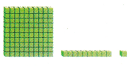to show the number two different ways. Draw both ways.

• What information am I given?
• How can I use pictorial models to show two different ways?

Question 1.Explanation:
Total number is blocks are 46
So we can write as 4 tens and 6 ones   = 3 tens and 16 ones = 46

Question 2.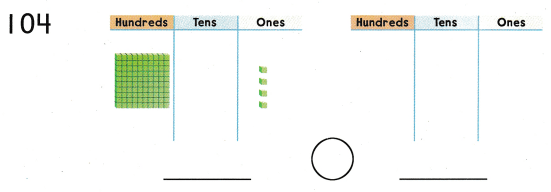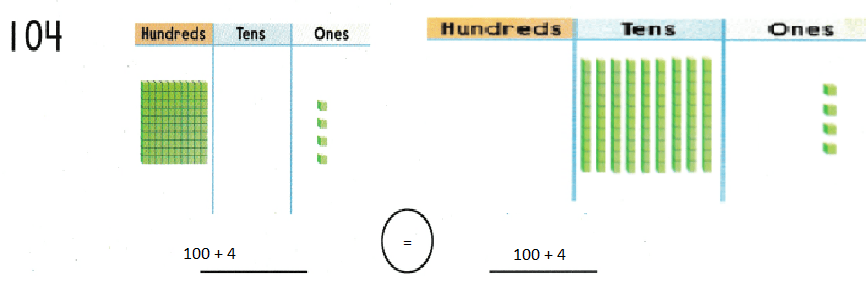Explanation:
Total number is blocks are 104
So we can write as 1 hundred and 4 ones  or 10 tens and 4 ones

Question 3.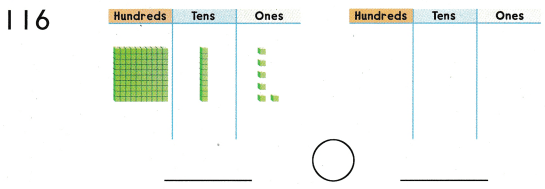Explanation:
Total number is blocks are 116
So we can write as 1 hundred 1 tens and 6 ones  or 10 tens and 16 ones

Math Talk
Mathematical Processes
Look at Exercise 3. Explain why both ways show 116.

Share and Show

Use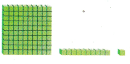to show the number two different ways. Draw both ways.

Question 4.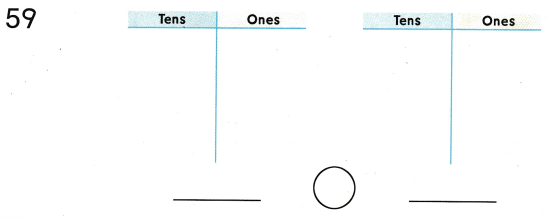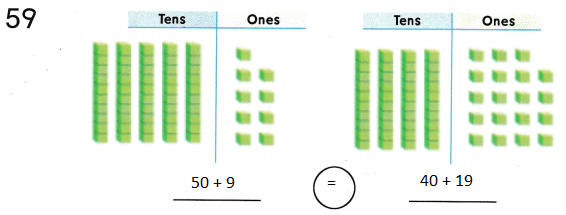Explanation:
Total number is blocks are 59
So we can write as 5 tens and 9 ones   = 4 tens and 19 ones = 59

Question 5.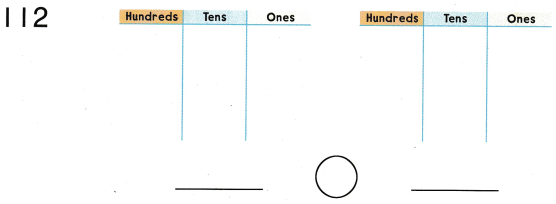Explanation:
Total number is blocks are 112
So we can write as 1 hundred 1 tens and 2 ones  or 10 tens and 12 ones

Problem Solving

Solve. Draw to Explain.

Question 6.
H.O.T. Multi-Step Kevin has 10 packs of cards. Aubrey has 2 packs of cards. There are 10 cards in each pack. How many cards do they have?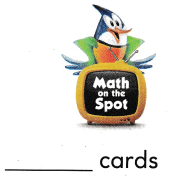Explanation:
There are 10 cards in each pack
Kevin has 10 packs of cards. = 10 tens = 100
Aubrey has 2 packs of cards. = 2 tens = 20
10 tens + 2 tens = 12 tens= 120

Question 7.
Analyze Tamra shows 36. Which is a different way to show the same number?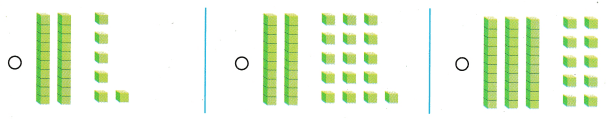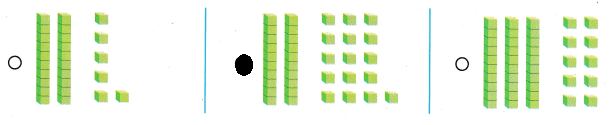Explanation:
Total number is blocks are 36
So we can write as 3 tens and 6 ones  or 2 tens and 16 ones

Question 8.
Bobby shows 44. Which is a different way to show the same number?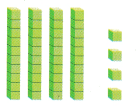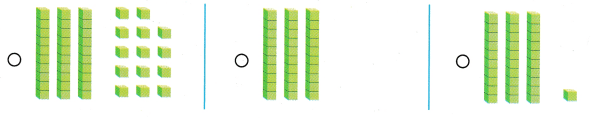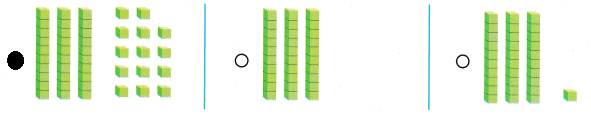Explanation:
Total number is blocks are 44
So we can write as 4 tens and 4 ones  or 3 tens and 14 ones

Question 9.
Texas Test Prep Use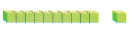to show the tens and ones. Which is a different way to show the same number?Explanation:
Total number is blocks are 23
So we can write as 1 tens and 13 ones  or 2 tens and 3 ones

Take Home Activity

• Have your child draw quick pictures to show the number 56 two ways.

### Texas Go Math Grade 1 Lesson 1.6 Homework and Practice Answer Key

Usethe number in two different ways. Draw both ways

Question 1.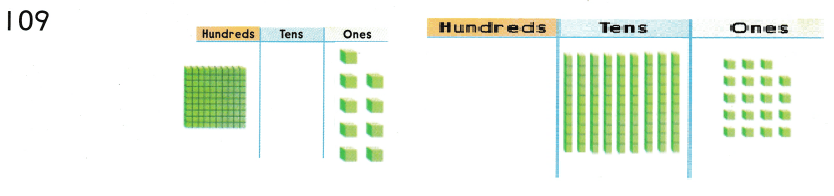Explanation:
Total number is blocks are 73
So we can write as 1 hundred and and 9 ones  or 9 tens and 19 ones

Question 2.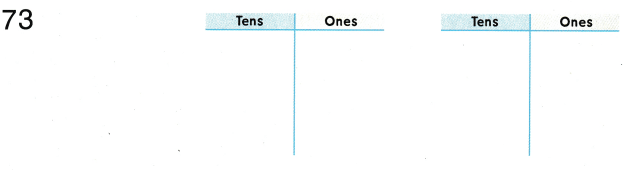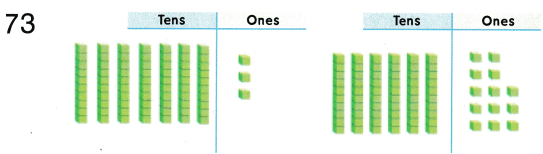Explanation:
Total number is blocks are 73
So we can write as 6 tens and 13 ones  or 7 tens and 3 ones

Problem Solving

Solve. Draw to explain.

Question 3.
Multi-Step Trish has 9 boxes of pencils. Raj has 2 boxes of pencils. There are 10 pencils in each box. How many pencils do they have?
____ pencils
Explanation:
There are 10 pencils in each box
Trish has 9 boxes of pencils.= 9 tens
Raj has 2 boxes of pencils = 2 tens
Total = 9 tens + 2 tens = 11 tens = 1 hundred and 1 ten= 110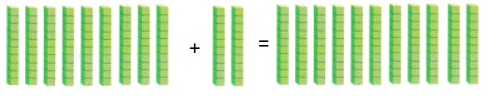Texas Test Prep

Lesson Check

Question 4.
Mr. Brown visits different states. He goes to 29 states. Which model shows 29?Explanation:
Explanation:
Total number is blocks are 29
So we can write as 1 tens and 19 ones.

Question 5.
Jennie picked 73 cherries from a tree. Which is a different way to show 73?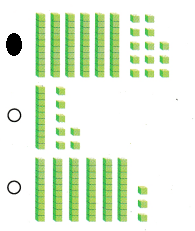Explanation:
Total number is blocks are 73
So we can write as 6 tens and 13 ones.

Question 6.
Which is a different way to show the same number?